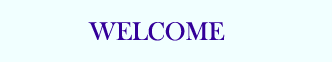TUTORIALS

DOS Quiz
DOS Exam

# Binary and hexadecimal conversions

Quick links

## Binary

Computers work on the principle of number manipulation. Inside the computer, the numbers are represented in bits and bytes. For example, the number three is represented by a byte with bits 0 and 1 set; 00000011. This is numbering system using base 2. People commonly use a decimal or Base 10 numbering system. What this means is that in Base 10, count from 0 to 9 before adding another digit. The number 22 in Base 10 means we have 2 sets of 10's and 2 sets of 1's.

Base 2 is also known as binary since there can only be two values for a specific digit; either a 0 = OFF or a 1 = ON. You cannot have a number represented as 22 in binary notation. The decimal number 22 is represented in binary as 00010110 which by following the below chart breaks down to:

 Bit Position 7 6 5 4 3 2 1 0 1 1 1 1 1 1 1 1 Decimal 128 64 32 16 8 4 2 1

22 or 00010110:

All numbers representing 0 are not counted, 128, 64, 32, 8, 1 because 0 represents OFF

However, numbers representing 1 are counted, 16 + 4 + 2 = 22 because 1 represents ON

Decimal Values and Binary Equivalents chart

 Decimal Binary 1 1 2 10 3 11 4 100 5 101 6 110 7 111 8 1000 9 1001 10 1010 16 10000 32 100000 64 1000000 100 1100100 256 100000000 512 1000000000 1000 1111101000 1024 10000000000

## Hexadecimal

The other major numbering system used by computers is hexadecimal, or Base 16. In this system, the numbers are counted from 0 to 9, then letters A to F before adding another digit. The letter A through F represent decimal numbers 10 through 15, respectively. The below chart indicates the values of the hexadecimal position compared to 16 raised to a power and decimal values. It is much easier to work with large numbers using hexadecimal values than decimal.

To convert a value from hexadecimal to binary, you merely translate each hexadecimal digit into its 4-bit binary equivalent. Hexadecimal numbers have either and 0x prefix or an h suffix. For example, the hexadecimal number:

0x3F7A

Translates into, Using the Binary chart and the below chart for Hex:

0011 1111 0111 1010

0 0 0000
1 1 0001
2 2 0010
3 3 0011
4 4 0100
5 5 0101
6 6 0110
7 7 0111
8 8 1000
9 9 1001
10 A 1010
11 B 1011
12 C 1100
13 D 1101
14 E 1110
15 F 1111

## Additional information:

• See the binary, hexadecimal, and octal definitions for further information about each of these terms and related links.